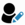## Search Java Code SnippetsHelp us in improving the repository. Add new snippets through 'Submit Code Snippet ' link.Submit Code Snippet

#Java - Code Snippets for '#Rounding a number' - 2 code snippet(s) foundSample 1. Round a Big Decimal Number with 5 precision and to upper value using MathContext``````MathContext mathContext = new MathContext(5,RoundingMode.HALF_UP);
System.out.println(new BigDecimal(100.4767,mathContext));``````LikeFeedbackrounding a number  BigDecimal  rounding Bigdecimal  RoundingMode  MathContext  RoundingMode.HALF_UPSample 2. Round a Big Decimal Number with 5 precision and to half lower value using MathContext``````MathContext mathContext = new MathContext(5,RoundingMode.HALF_DOWN);
System.out.println(new BigDecimal(100.4767,mathContext));``````LikeFeedbackrounding a number  BigDecimal  rounding Bigdecimal  RoundingMode  MathContext  RoundingMode.HALF_DOWN

Subscribe to Java News and Posts. Get latest updates and posts on Java from Buggybread.com